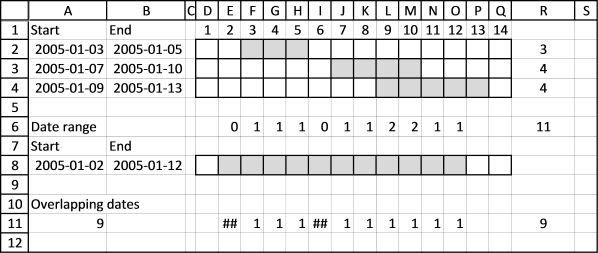Author: Oscar Cronquist Article last updated on October 09, 2018

Thanks a tonne, Oscar. It took me about 8 hours to work through this formula piece by piece, play with it, and come to grips with its basics. Your example was clear and very useful, and this has allowed me to do big, very useful data analysis for the company that employs me. It applies to thousands of people. You're an unsung hero.

Actually just realized that I really need what you said you'd "save for a future post" (actually total number of overlapping dates). I guess I'll try to figure that out. Still... couldn't have gotten this close without you.

Thanks NC, here comes that "future" post. This post demonstrates how to count overlapping days except duplicates across multiple date ranges.I began working with this formula in cell range R2:R4:

=MMULT((TRANSPOSE(\$A\$8+ROW(A1:INDEX(\$A:\$A, \$B\$8-\$A\$8+1))-1)>=\$A\$2:\$A\$4)*(TRANSPOSE(\$A\$8+ROW(A1:INDEX(\$A:\$A, \$B\$8-\$A\$8+1))-1)<=B2:B4), ROW(A1:INDEX(\$A:\$A, \$B\$8-\$A\$8+1))^0)

This formula returns a number indicating overlapping days, for each date range. There are 3 date ranges so the array contains 3 values.

### Modified formula

What I was looking for was a formula that counts overlapping days, for each date instead of each date range. The date range is 2005-01-02 / 2005-01-12, 11 days and therefore 11 values in the returning array. This is what I came up with, entered in cell range E6:O6:

=MMULT(TRANSPOSE(B2:B4^0), (TRANSPOSE(\$A\$8+ROW(A1:INDEX(\$A:\$A, \$B\$8-\$A\$8+1))-1)>=\$A\$2:\$A\$4)*(TRANSPOSE(\$A\$8+ROW(A1:INDEX(\$A:\$A, \$B\$8-\$A\$8+1))-1)<=B2:B4))

You can´t sum the values in this array, you will get 11, counting duplicates that I don´t want. (0+1+1+1+0+1+1+2+2+1+1 = 11).

### Convert values in array

This array formula, entered in E8:O8, converts zeros to #num error and values above 0 to 1.

=MMULT(TRANSPOSE(B2:B4^0), (TRANSPOSE(\$A\$8+ROW(A1:INDEX(\$A:\$A, \$B\$8-\$A\$8+1))-1)>=\$A\$2:\$A\$4)*(TRANSPOSE(\$A\$8+ROW(A1:INDEX(\$A:\$A, \$B\$8-\$A\$8+1))-1)<=B2:B4))^0

returns

{#NUM!, 1, 1, 1, #NUM!, 1, 1, 1, 1, 1, 1}

### Count number of values in array that contain numbers

=COUNT(MMULT(TRANSPOSE(B2:B4^0), (TRANSPOSE(\$A\$8+ROW(A1:INDEX(\$A:\$A, \$B\$8-\$A\$8+1))-1)>=\$A\$2:\$A\$4)*(TRANSPOSE(\$A\$8+ROW(A1:INDEX(\$A:\$A, \$B\$8-\$A\$8+1))-1)<=B2:B4))^0)

returns 9.

This array formula is entered in cell A11.